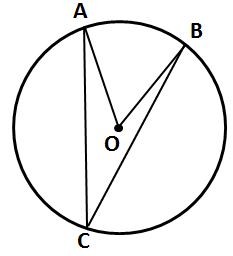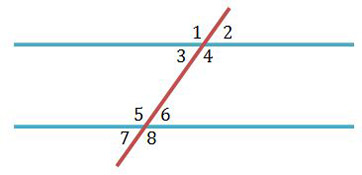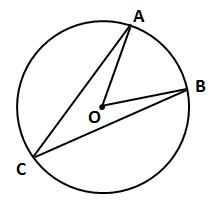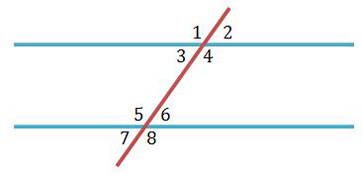NY Regents Exam - Geometry: Help and Review Final Exam

Free Practice Test Instructions:

Choose your answer to the question and click 'Continue' to see how you did. Then click 'Next Question' to answer the next question. When you have completed the free practice test, click 'View Results' to see your results. Good luck!

Question 5 5. If angle AOB is 40 degrees, what is the measure of angle ACB?Question 12 12. Which angle is equal to angle 1?Question 14 14. If the measure of central angle AOB is 62 degrees, what is the measure of inscribed angle ACB?Question 15 15. If angle 2 measures 60, what does angle 8 measure if the lines are parallel?